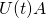### Left circular orbits in SU(1,1)

We continue, from the previous post, with the beauty of the group SU(1,1). We already know that it looks like a doughnut, it feels like a doughnut, and it tastes like a doughnut. Yummy.We represented SU(1,1) as a product of the open unit disk D and the circle parametrizing it byand. The circle group U(1) can be considered as a subgroup of SU(1,1). We analyzed orbits of the right action of U(1) on SU(1,1) using the (parametrization:The orbits are circles going around the doughnut. Every point ofdetermines one orbit.

What about orbits of the left action? The truth is: we will not need them in what follows, but it is natural question to ask, so why not to ask?

So, let us takeof the form

(1)We takein SU(1,1), represent it as, and we contemplate the product– withfixed andrunning fromto, we get one orbit. In order to represent the points of this orbit in terms of parametersandwe need to representasWe do it by using a little trick, one of the standard tricks that every magician knows. We write

(2)Here we used the fact thatare unitary,and that they have the group propertyFrom the fact thatare unitary follows even more.is Hermitian, thereforeis also Hermitian. Moreover,has the same eigenvalues asSinceis positive, thereforeis also positive, and it is also an an element of SU(1,1), therefore it must be of the formfor some. By direct calculation we find that

(3)Now, sincewe deduce thatTherefore the orbit is a path around the donut that, while going around, moves on a circle around the center of the disk – the cross-section of the donut. While going once around the donut, it makes two full circles in the disk – a closed spiral.

The centers of the disks form a separate orbit. It is the orbit of both right and left action. The centers of the disks correspond to